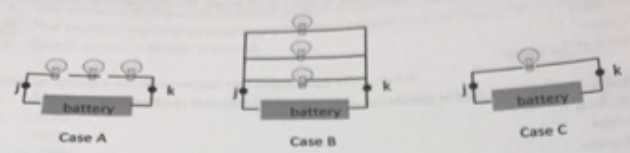# Problem: From lowest to highest, rank the current in the main branch of the circuit – that is – rank the current passing through point j. a. A, B, C b. A, C, B c. B, A, C d. B, C, A e. C, A, B f. C, B, A

###### FREE Expert Solution

Ohm's law:

$\overline{){\mathbit{i}}{\mathbf{=}}\frac{\mathbf{V}}{\mathbf{R}}}$

The bulbs act as resistors.

Assuming that each bulb has a resistance of R, we can get the equivalent resistance for the different cases from where we can get current.

Remember,

Equivalent resistance for resistors in parallel:

$\overline{)\frac{\mathbf{1}}{{\mathbf{R}}_{\mathbf{eq}}}{\mathbf{=}}\frac{\mathbf{1}}{{\mathbf{R}}_{\mathbf{1}}}{\mathbf{+}}\frac{\mathbf{1}}{{\mathbf{R}}_{\mathbf{2}}}{\mathbf{+}}{\mathbf{.}}{\mathbf{.}}{\mathbf{.}}{\mathbf{+}}\frac{\mathbf{1}}{{\mathbf{R}}_{\mathbf{n}}}}$

Equivalent resistance for resistors in series:

$\overline{){{\mathbf{R}}}_{{\mathbf{eq}}}{\mathbf{=}}{{\mathbf{R}}}_{{\mathbf{1}}}{\mathbf{+}}{{\mathbf{R}}}_{{\mathbf{2}}}{\mathbf{+}}{\mathbf{.}}{\mathbf{.}}{\mathbf{.}}{\mathbf{+}}{{\mathbf{R}}}_{{\mathbf{n}}}}$

97% (253 ratings)###### Problem DetailsFrom lowest to highest, rank the current in the main branch of the circuit – that is – rank the current passing through point j.

a. A, B, C

b. A, C, B

c. B, A, C

d. B, C, A

e. C, A, B

f. C, B, A

Frequently Asked Questions

What scientific concept do you need to know in order to solve this problem?

Our tutors have indicated that to solve this problem you will need to apply the Resistors and Ohm's Law concept. You can view video lessons to learn Resistors and Ohm's Law. Or if you need more Resistors and Ohm's Law practice, you can also practice Resistors and Ohm's Law practice problems.